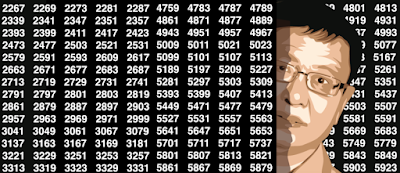## Friday, April 27, 2018

### New Decimal Systems - Great Sandbox for Data Scientists and Mathematicians

We illustrate pattern recognition techniques applied to an interesting mathematical problem: The representation of a number in non-conventional systems, generalizing the familiar base-2 or base-10 systems. The emphasis is on data science rather than mathematical theory, and the style is that of a tutorial, requiring minimum knowledge in mathematics or statistics. However, some off-the-beaten-path, state-of-the-art number theory research is discussed here, in a way that is accessible to college students after a first course in statistics. This article is also peppered with mathematical and statistical oddities, for instance the fact that there are units of information smaller than the bit.
You will also learn how the discovery process works, as I have included research that I thought would lead me to interesting results, but did not. In all scientific research, only final, successful results are presented, while actually most of the research leads to dead-ends, and is not made available to the reader. Here is your chance to discover these hidden steps, and my thought process!The topic discussed is one of active research with applications to Blockchain or strong encryption. It is of interest to agencies such as the NSA or private research labs working on security issues, which explains why it is not easy to find many references; some are probably classified documents. As far as I know, it is not part of any university curriculum either. Indeed, the fear of reversibility (successful attacks on cryptographic keys using modern computer networks, new reverse-engineering algorithms, and distributed architecture) has led to the development of quantum algorithms and quantum computers, as well as Blockchain.
All the results in this article were obtained without writing a single line of code, and replicable as I share my Excel spreadsheets.
Content of the article
1. General Framework
• Components of number representation systems
• General properties of these systems
• Examples of number representation systems
• Examples of patterns in digit distribution
• Purpose of this research
2. Defects found in the Logistic Map system
3. First step in designing a new system
• First version of new number representation system
• Holes, autocorrelations, and entropy (information theory)
4. Towards a more general, better, hybrid system
• Faulty digits, ergodicity, and high precision computing
• Finding the equilibrium distribution with the percentile test
• Central limit theorem, random walks, Brownian motions
• Data set and Excel computations
5. Related articles